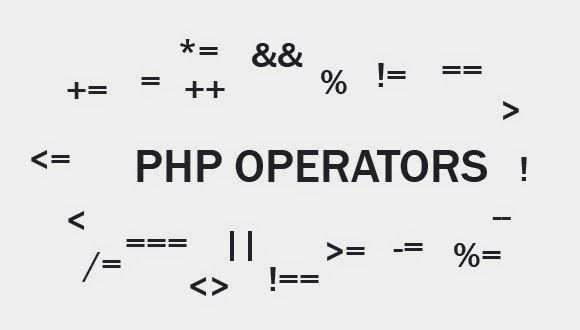# 运算符类型

PHP 语言支持以下类型的运算符。.

• 算术运算符
• 比较运算符
• 逻辑(或关系) 运算符
• 赋值运算符
• 条件赋值操作符(或三元) 运算符## 算术运算符

PHP 语言支持有以下算术运算符：

+ 变量 A 和变量 B 相加 A + B 等于 30

* 变量 A 和变量 B 相乘 A * B等于 200
/ 变量 A 和变量 B 相除 B / A等于2
% 变量 A 和变量 B 取余数 B % A等于0
++ 变量A++ A++等于11

## 比较运算符

== 检查两个操作数的值是否相等,如果相等,那么条件变为真。 (A == B) 是不为真的.
!= 检查两个操作数的值是否相等,如果值不相等,那么条件变为真。 (A != B) 为真.
> 检查左操作数的值是否大于右操作数的值,如果是的,那么条件变为真。 (A > B) 为假.
< 检查左操作数的值小于右操作数的值,如果是的,那么条件变为真。 (A
>= 检查左操作数的值是否大于或等于右操作数的值,如果是的,那么条件变为真。 (A >= B) 为假.
<= 检查左操作数的值是否小于或等于右操作数的值,如果是的,那么条件变为真。 (A

## 逻辑运算符:

and 称为逻辑与操作。如果两个操作数都是真的,那么条件变成真。 (A and B) 为真.
or 称为逻辑或运算符。如果两个操作数的任何一个非零,那么情况就变为真。 (A or B) 为真.
&& 称为逻辑与操作。如果两个操作数都非零然后条件变成真。 (A && B) 为真.
|| 称为逻辑或运算符。如果两个操作数的都不为零,那么情况就变为真。 (A || B) 为真。
! 称为逻辑非。使用反转逻辑状态的操作数。如果是真的,那么一个条件逻辑操作符将是假的。 !(A && B) 为假.

## 赋值运算符

= 简单的赋值运算符,将来自右操作数的值赋给左边的操作数 C = A + B 将赋值给C赋值 A + B
+= 添加和赋值运算符,它将右操作数与左操作数的求和结果分配给左操作数 C += A 等于 C = C + A
-= 减去和赋值运算符,右操作数减去左操作数,并将结果分配给左操作数 C -= A 等于 C = C – A
*= 相乘和赋值运算符,它可以使右操作数乘以左操作数,并将结果分配给左操作数 C *= A 等于 C = C * A
/= 相除和赋值运算符,将左操作数除以右操作数,并将结果分配给左操作数 C /= A 等于 C = C / A
%= 取模和赋值运算符, 将左操作数除以右操作数的余数分配到左操作数的结果 C %= A 等于 C = C % A

## 条件运算符

? : 条件表达式 条件为真则返回？号后面的值否则返回：号后面的值

## 运算符分类

• 一元前缀运算符，优先操作一个操作数。
• 二元运算符，这两个操作数，执行各种算术和逻辑操作。
• 条件操作符(三元操作符)，将三个操作数，计算第二个或第三个表达式，根据评估的第一个表达式。
• 赋值操作符，将值分配给一个变量。

## 运算符优先级

Unary ! ++ — 从右往左
Multiplicative * / % 从左向右
Additive + – 从左向右
Relational < <= > >= 从左向右
Equality == != 从左向右
Logical AND && 从左向右
Logical OR || 从左向右
Conditional ?: 从右往左
Assignment = += -= *= /= %= 从右往左

Posted in PHP教程